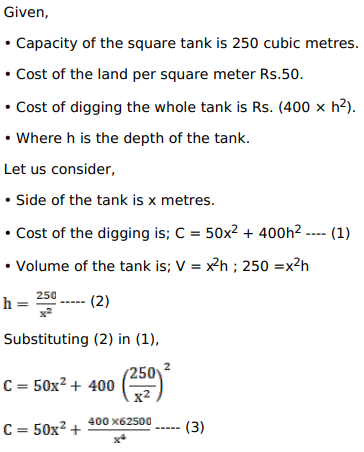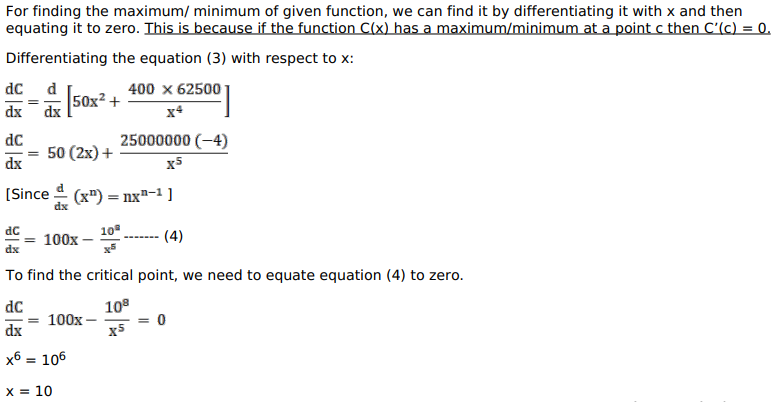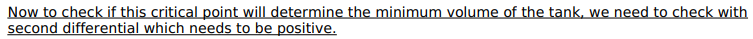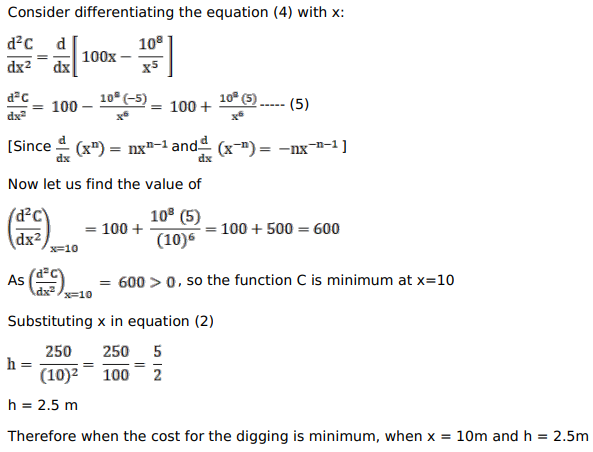# A square tank of capacity 250 cubic meters has to be dug out.

Question:

A square tank of capacity 250 cubic meters has to be dug out. The cost of the land is Rs. 50 per square metre. The cost of digging increases with the depth and for the whole tank, it is Rs. $\left(400 \times h^{2}\right)$, where $h$ metres is the depth of the tank. What should be the dimensions of the tank so that the cost is minimum?

Solution: